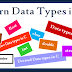The data types in C tells the compiler or interpreter what type of data a programmer is using and how many memory (bytes) a variable is needed. How much memory a variable is needed is predefined. Data types are Primary data types and Derived data types.

### Pre-define Data Types or Fundamental Data Types in C

This data type was defined by C standard and these data types are int (integer), float (floating), char (character), double etc. With the help of the sizeof operator, we can know the size of data types in C. These data types are the platform dependent so, C standard also introduces the fixed size of data type like uint8_t, uint16_t etc. Pre-define data types are defined in <stdint.h> header file. These data types are also known as primary data types in C.

#### int

int is a whole number, it keeps both zero, negative and positive numbers, it does not contain any decimal value.
Syntax
int name_of_variable;

Size of an integer is 4 bytes for the 32-bit compiler.

#### float

It stores decimal values with single accuracy. Float is used to hold real numbers. Floating point numbers can also be represented exponentially (Example: 25.936e5).
Syntax
float price_of_product;

#### char

This data type is used to define the character variable and it needs one byte of memory.
Syntax
char name_of_character;
char a = 'c';

#### double

It stores decimal values with double accuracy. Double is also used to hold real numbers.
Syntax
double salary;

#### Signed and unsigned

Signed and unsigned are type modifiers. With the help of these modifiers, we can change the data storage of a data type. For example,
int a;
unsigned int b;

Here, the variable 'a' can hold positive and negative values, and its range is from -2^31 to 2^31-1. Whereas variable 'b' can hold zero and positive values, and its range is from 0 to 2^32-1.

Each data types has its own range of how many numbers or values it can store. These ranges vary from compiler to compiler (like 32-bit compiler have different range and 64-bit compiler have the different range).

Below a program has been written using the sizeof operator, with the help of sizeof operator you can learn how to find out the size of different data types in C.

#include <stdio.h>
int main( )
{
int number = 20;
char alphabet = 'A';
float d = 8.6;
double c = 7.22;
printf ("Welcome\n");

//Printing the value and size of an integer data type.
printf ("Welcome, I am an integer. My value is %d and size is %lu bytes.\n", number, sizeof (number));

//Printing the value and size of a character data type.
printf ("Welcome, I am a character. My value is %c and size is %lu bytes.\n", alphabet, sizeof (alphabet));

//Printing the value and size of float data types in C
printf ("Welcome, I am float. My value is %f and size is %lu bytes. \n",d,sizeof (d));

//Printing the value and size of double data types in C
printf ("Welcome, I am double. My value is %lf and size is %lu bytes. \n", c, sizeof (c));

printf ("Goodbye");
return 0;
}

Output
Welcome
Welcome, I am an integer. My value is 20 and size is 4 bytes.
Welcome, I am a character. My value is A and size is 1 byte.
Welcome, I am float. My value is 8.600000 and size is 4 bytes.
Welcome, I am double. My value is 7.220000 and size is 8 bytes.
Goodbye

### User-define data types in C

C language allows programmers to create their own data type. A user-defined data type is created by the user by using the keyword struct (Structure in C), union (Union in C), array (Array in C), strings (Strings in C), Function, pointer, enum etc.

Learn Data Types in CReviewed by Glory on 21:45 Rating: 5